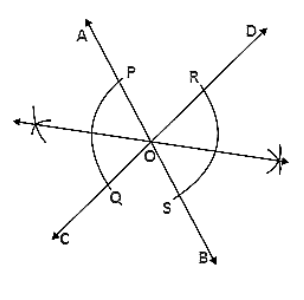# Draw a pair of vertically opposite angles.

Question:

Draw a pair of vertically opposite angles. Bisect each of the two angles. Verify that the bisecting rays are in the same line.

Solution:

Steps of Construction:

1. Draw a pair of vertically opposite angle ∠AOC and ∠DOB.

2. Keeping O as the center and any radius draw two arcs which intersect OA at P, OC at Q, OB at S and OD at R.

3. Keeping P and Q as center and radius more than half of PQ draw two arcs which intersect each other at T.

4. Join TO.

5. Keeping R and S as center and radius more than half of RS draw two arcs which intersect each other at U.

6. Join OU.

Therefore TOU is a straight line